# Optimal and Fair Spatial Siting

A bit of a belated MLK day post. Much of the popular news on predictive or machine learning algorithms has a negative connotation, often that they are racially biased. I tend to think about algorithms though in almost the exact opposite way – we can adjust them to suit our objectives. We just need to articulate what exactly we mean by fair. This goes for predictive policing (Circo & Wheeler, 2021; Liberatore et al., 2021; Mohler et al., 2018; Wheeler, 2020) as much as it does for any application.

I have been reading a bit about spatial fairness in siting health resources recently, one example is the Urban Institutes Equity Data tool. For this tool, you put in where your resources are currently located, and it tells you whether those locations are located in areas that have demographic breakdowns like the overall city. So this uses the container approach (not distance to the resources), which distance traveled to resources is probably a more typical way to evaluate fair spatial access to resources (Hassler & Ceccato, 2021; Koschinsky et al., 2021).

Here what I am going to show is instead of ex-ante saying whether the siting of resources is fair, I construct an integer linear program to site resources in a way we define to be fair. So imagine that we are siting 3 different locations to do rapid Covid testing around a city. Well, we do the typical optimization and minimize the distance traveled for everyone in the city on average to those 3 locations – on average 2 miles. But then we see that white people on average travel 1.9 miles, and minorities travel 2.2 miles. So it that does not seem so fair does it.

I created an integer linear program to take this difference into account, so instead of minimizing average distance, it minimizes:

``White_distance + Minority_distance + |White_distance - Minority_distance|``

So in our example above, if we had a solution that was white travel 2.1 and minority 2.1, this would be a lower objective value than (4.2), than the original minimize overall travel (1.9 + 2.2 + 0.3 = 4.4). So this gives each minority groups equal weight, as well as penalizes if one group (either whites or minorities) has much larger differences.

I am not going to go into all the details. I have python code that has the functions (it is very similar to my P-median model, Wheeler, 2018). The codes shows an example of siting 5 locations in Dallas (and uses census block group centroids for the demographic data). Here is a map of the results (it has points outside of the city, since block groups don’t perfectly line up with the city boundaries).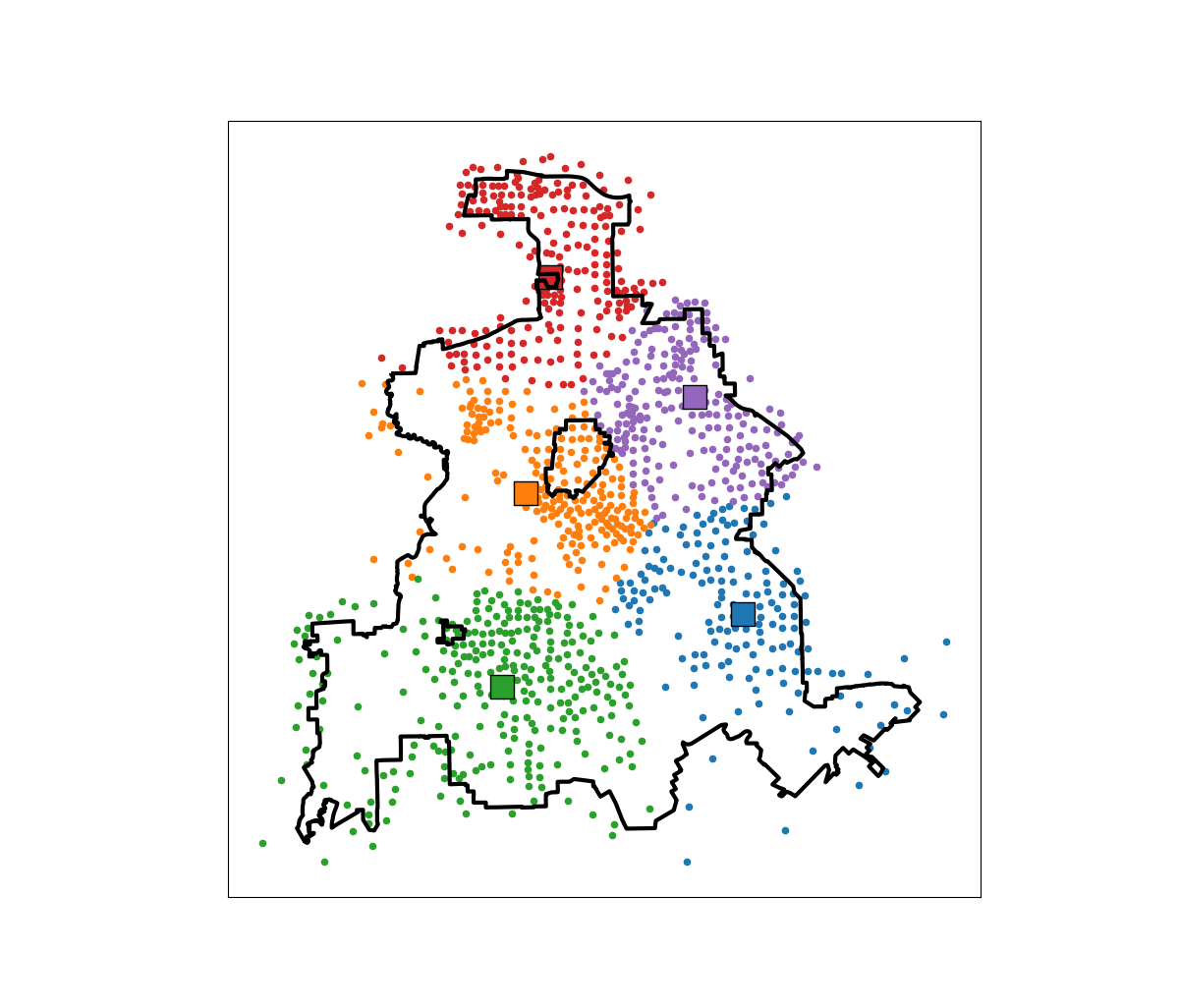In this example, if we choose 5 locations in the city to minimize the overall distance, the average travel is just shy of 3.5 miles. The average travel for white people (not including Hispanics) is 3.25 miles, and for minorities is 3.6 miles. When I use my fair algorithm, the white average distance is 3.5 miles, and the minority average distance is 3.6 miles (minority on average travels under 200 more feet on average than white).

So this is ultimately a trade off – it ends up pushing up the average distance a white person will travel, and only slightly pushes down the minority travel, to balance the overall distances between the two groups. It is often the case though that one can be somewhat more fair, but in only results in slight trade-offs though in the overall objective function (Rodolfa et al., 2021). So that trade off is probably worth it here.

# Learning a fair loss function in pytorch

Most of the time when we are talking about deep learning, we are discussing really complicated architectures – essentially complicated sets of (mostly linear) equations. A second innovation in the application of deep learning though is the use of back propagation to fit under-determined systems. Basically you can feed these systems fairly complicated loss functions, and they will chug along with no problem. See a prior blog post of mine of creating simple regression weights for example.

Recently in the NIJ challenge, I used pytorch and the non-linear fairness function defined by NIJ. In the end me and Gio used an XGBoost model, because the data actually do not have very large differences in false positive rates between racial groups. I figured I would share my example here though for illustration of using pytorch to learn a complicated loss function that has fairness constraints. And here is a link to the data and code to follow along if you want.

First, in text math the NIJ fairness function was calculated as `(1 - BS)*(1 - FP_diff)`, where BS is the Brier Score and FP_diff is the absolute difference in false positive rates between the two groups. My pytorch code to create this loss function looks like this (see the `pytorch_mods.py` file):

``````# brier score loss function with fairness constraint
def brier_fair(pred,obs,minority,thresh=0.5):
bs = ((pred - obs)**2).mean()
over = 1*(pred > thresh)
majority = 1*(minority == 0)
fp = 1*over*(obs == 0)
min_tot = (over*minority).sum().clamp(1)
maj_tot = (over*majority).sum().clamp(1)
min_fp = (fp*minority).sum()
maj_fp = (fp*majority).sum()
min_rate = min_fp/min_tot
maj_rate = maj_fp/maj_tot
diff_rate = torch.abs(min_rate - maj_rate)
fin_score = (1 - bs)*(1 - diff_rate)
return -fin_score``````

I have my functions all stashed in different py files. But here is an example of loading up all my functions, and fitting my pytorch model to the training recidivism data. Here I set the threshold to 25% instead of 50% like the NIJ competition. Overall the model is very similar to a linear regression model.

``````import data_prep as dp
import fairness_funcs as ff
import pytorch_mods as pt

# Get the train/test data
train, test = dp.get_y1_data()
y_var = 'Recidivism_Arrest_Year1'
min_var = 'Race' # 0 is White, 1 is Black
x_vars = list(train)
x_vars.remove(y_var)

# Model learning fair loss function
m2 = pt.pytorchLogit(loss='brier_fair',activate='ident',
minority=min_var,threshold=0.25)
m2.fit(train[x_vars],train[y_var])``````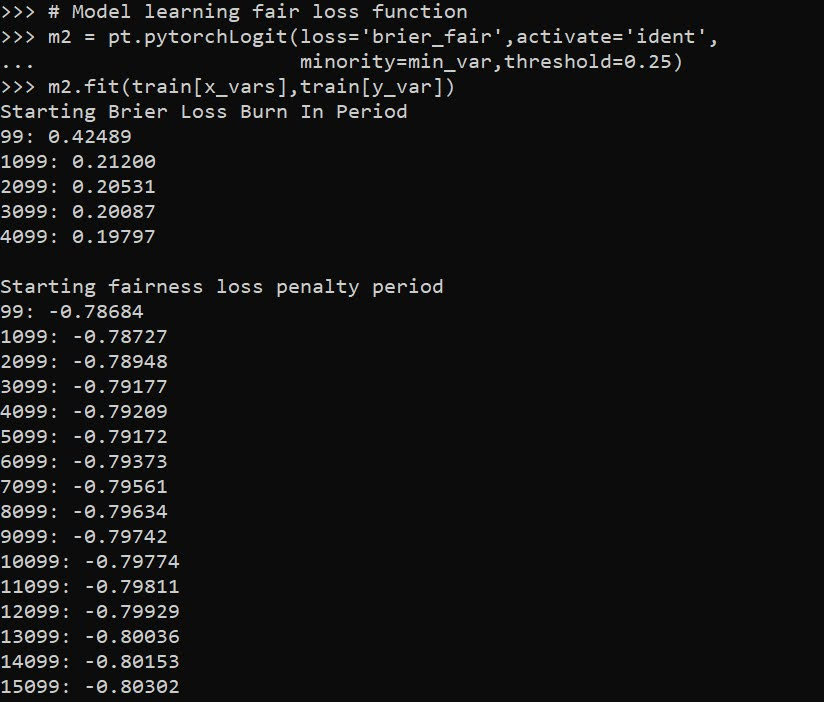I have a burn in start to get good initial parameter estimates with a more normal loss function before going into the more complicated function. Another approach would be to initialize the weights to the solution for a linear regression equation though. After that burn in though it goes into the NIJ defined fairness loss function.

Now I have functions to see how the different model metrics in whatever sample. Here you can see the model is quite balanced in terms of false positive rates in the training sample:

``````# Seeing training sample fairness
m2pp = m2.predict_proba(train[x_vars])[:,1]
ff.fairness_metric(m2pp,train[y_var],train[min_var],thresh=0.25)``````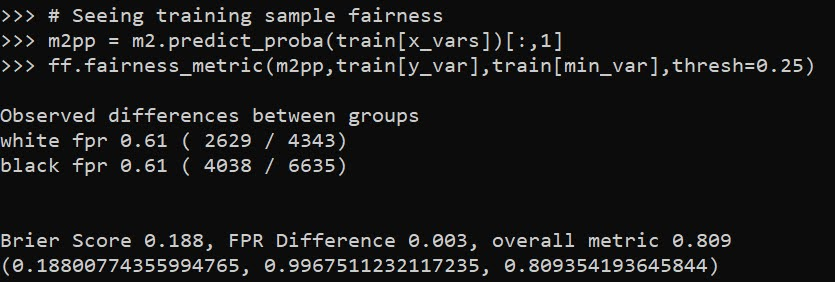But of course in the out of sample test data it is not perfectly balanced. In general you won’t be able to ensure perfect balance in whatever fairness metrics out of sample.

``````# Seeing test sample fairness
m2pp = m2.predict_proba(test[x_vars])[:,1]
ff.fairness_metric(m2pp,test[y_var],test[min_var],thresh=0.25)``````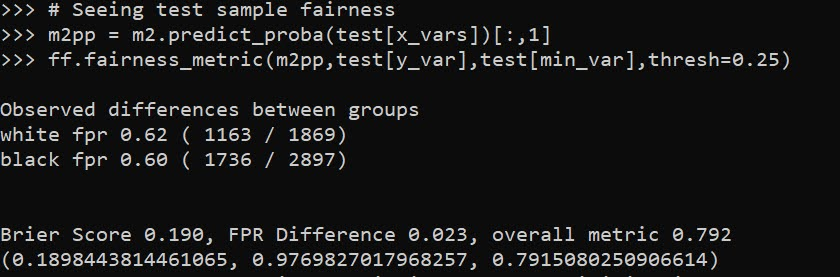It actually ends up that the difference in false positive rates between the two racial groups, even in models that do not incorporate the fairness constraint in the loss function, are quite similar. Here is a model using the same architecture but just the usual Brier Score loss. (Code not shown, see the `m1` model in the `01_AnalysisFair.py` file in the shared dropbox link earlier.)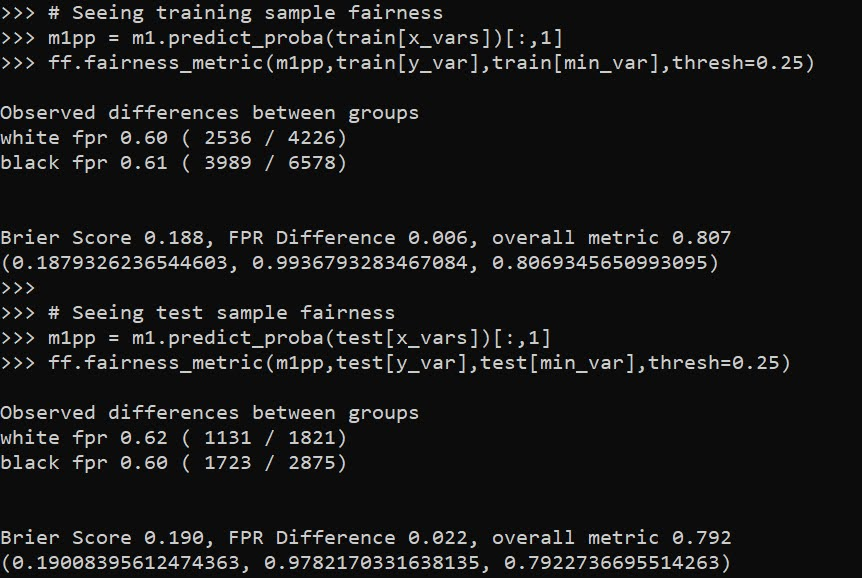You can read mine and Gio’s paper (or George Mohler and Mike Porter’s paper) about why this particular fairness function is not the greatest. In general it probably makes more sense to use an additive fairness loss instead of multiplicative, but in this dataset it won’t matter very much no matter how you slice it in terms of false positive rates. (It appears in retrospect the Compas data that started the whole false positive thing is somewhat idiosyncratic.)

There are other potential oddities that can occur with such fair loss functions. For example if you had the choice between false positive rates for `(white,black)` of `(0.62,0.60)` vs `(0.62,0.62)`, the latter is more fair, but the minority class is worse off. It may make more sense to have an error metric that sets the max false positive rate you want, and then just has weights for different groups to push them down to that set threshold.

These aren’t damning critiques of fair loss functions (these can be amended/changed to behave better), but in the end defining fair loss functions will be very tricky. Both for empirical reasons as well as for ethical ones – they will ultimately involve quite a few trade-offs.

# Balancing False Positives

One area of prediction in criminal justice I think has alot of promise is using predictive algorithms in place of bail decisions. So using a predictive instrument to determine whether someone is detained pre-trial based on risk, or released on recognizance if you are low risk. Risk can be either defined as based on future dangerousness or flight risk. This cuts out the middle man of bail, which doesn’t have much evidence of effectiveness, and has negative externalities of placing economic burdens on folks we really don’t want to pile that onto. It is also the case algorithms can likely do quite a bit better than judges in figuring out future risk. So an area I think they can really do good compared to current status quo in the CJ system.

A reasonable critique of such systems though is they can have disparate racial impact. For example, ProPublica had an article on how the Compas risk assessment instrument resulted in more false positives for black than white individuals. Chris Stucchio has a nice breakdown for why this occurs, which is not due to the Compas being intrinsically racist algorithm, but due to the nature of the baseline risks for the two groups.

Consider a very simple example to illustrate. Imagine based on our cost-benefit analysis, we determine the probability threshold to flag a individual as high risk is 60%. Now say our once we apply our predictions, for those above the threshold, whites are all predicted to be 90%, and blacks are all 70%. If our model is well calibrated (which is typically the case), the false positive rate for whites will be 10%, and will be 30% for blacks.

It is actually a pretty trivial problem though to balance false positive rates between different groups, if that is what you want to do. So I figured I would illustrate here using the same ProPublica data. There are trade-offs though with this, balancing false positives means you lose out on other metrics of fairness. In particular, it means you don’t have equality of treatment – different racial groups will have different thresholds. The full data and code I use to illustrate this can be downloaded here.

## An Example in Python

To illustrate how we would balance the false positive rates between groups, I use the same ProPublica risk assessment data. So this isn’t per se for bail decisions, but works fine as an illustration. First in python I load my libraries, and then read in the data – it is a few over 11,000 cases.

``````import pandas as pd
import os
import numpy as np
from sklearn.ensemble import RandomForestClassifier
import matplotlib.pyplot as plt

my_dir = r'C:\Users\andre\Dropbox\Documents\BLOG\BalanceFalsePos'
os.chdir(my_dir)

#For notes on data source, check out
#https://github.com/apwheele/ResearchDesign/tree/master/Week11_MachineLearning

Next I prepare the dataset for modelling. I am not using all of the variables in the dataset. What I predict here is recidivism post 30 days (there are a bunch of recidivism right away in the dataset, so I am not 100% sure those are prior to screening). I use the three different aggregate compas scores, juvenile felony count, whether they were male, how old they were, and whether the current charge to precipitate screening is a felony or misdemeanor. I include the race variable in the dataset, but I won’t be using it in the predictive model. (That point deserves another blog post, contra to what you might expect, leaving race flags in will often result in better outcomes for that protected class.)

``````#Preparing the variables I want
recid_prep = recid[['Recid30','CompScore.1','CompScore.2','CompScore.3',
recid_prep['Male'] = 1*(recid['sex'] == "Male")
recid_prep['Fel'] = 1*(recid['c_charge_degree'] == "F")
recid_prep['Mis'] = 1*(recid['c_charge_degree'] == "M")
recid_prep['race'] = recid['race']
print( recid['race'].value_counts() ) #pretty good sample size for both whites/blacks``````

Next I make my testing and training sets of data. In practice I can perfectly balance false positives retrospectively. But having a test set is a better representation of reality, where you need to make some decisions on the historical data and apply it forward.

``````#Now generating train and test set
recid_prep['Train'] = np.random.binomial(1,0.75,len(recid_prep))
recid_train = recid_prep[recid_prep['Train'] == 1]
recid_test = recid_prep[recid_prep['Train'] == 0]``````

Now the procedure I suggest to balance false-positives doesn’t matter how you generate the predictions, just that we need a predicted probability. Here I use random forests, but you could use whatever machine learning or logistic regression model you want. Second part just generates the predicted probabilities for the training dataset.

``````#Now estimating the model
ind_vars = ['CompScore.1','CompScore.2','CompScore.3',
dep_var = 'Recid30'
rf_mod = RandomForestClassifier(n_estimators=500, random_state=10)
rf_mod.fit(X = recid_train[ind_vars], y = recid_train[dep_var])

#Now getting the predicted probabilities in the training set
pred_prob = rf_mod.predict_proba(recid_train[ind_vars] )
recid_train['prob'] = pred_prob[:,1]
recid_train['prob_min'] = pred_prob[:,0]``````

Now to balance false positives, I will show a graph. Basically this just sorts the predicted probabilities in descending order for each racial group. Then you can calculate a cumulate false positive rate for different thresholds for each group.

``````#Making a cusum plot within each racial group for the false positives
recid_train.sort_values(by=['race','prob'], ascending=False, inplace=True)
recid_train['const'] = 1
recid_train['cum_fp'] = recid_train.groupby(['race'])['prob_min'].cumsum()
recid_train['cum_n'] = recid_train.groupby(['race'])['const'].cumsum()
recid_train['cum_fpm'] = recid_train['cum_fp'] / recid_train['cum_n']
white_rt = recid_train[recid_train['race'] == 'Caucasian']
black_rt = recid_train[recid_train['race'] == 'African-American' ] ``````

And now the fun part (and least in output, not really in writing matplotlib code).

``````#now make the chart for white and black
fig, ax = plt.subplots()
ax.plot(black_rt['prob'], black_rt['cum_fpm'], drawstyle='steps', color='b', label='Black')
ax.plot(white_rt['prob'], white_rt['cum_fpm'], drawstyle='steps', color='r', label='White')
ax.set_xlim(1, 0)  # decreasing probs
plt.xticks(np.arange(1.0,-0.1,-0.1))
ax.set_xlabel('Predicted Probability')
ax.set_ylabel('Mean False Positive Rate')
ax.grid(True,linestyle='--')
ax.legend(facecolor='white', framealpha=1)
plt.savefig('FP_Rate.png', dpi=2000, bbox_inches='tight')
plt.show()``````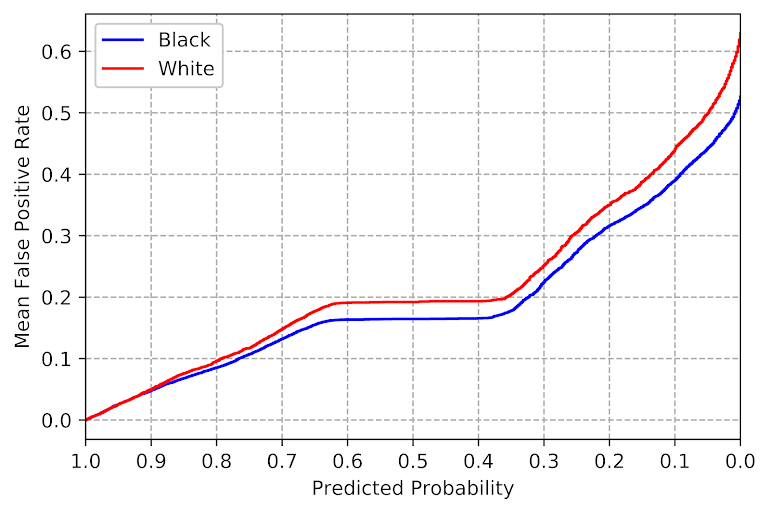So what this chart shows is that if we set our threshold to a particular predicted probability (X axis), based on the data we would expect a false positive rate (Y axis). Hence if we want to balance false positives, we just figure out the race specific thresholds for each group at a particular Y axis value. Here we can see the white line is actually higher than the black line, so this is reverse ProPublica findings, we would expect whites to have a higher false positive rate than blacks given a consistent predicted probability of high risk threshold. So say we set the threshold at 10% to flag as high risk, we would guess the false positive rate among blacks in this sample should be around 40%, but will be closer to 45% in the white sample.

Technically the lines can cross at one or multiple places, and those are places where you get equality of treatment and equality of outcome. It doesn’t make sense to use that though from a safety standpoint – those crossings can happen at a predicted probability of 99% (so too many false negatives) or 0.1% (too many false positives). So say we wanted to equalize false positive rates at 30% for each group. Here this results in a threshold for whites as high risk of 0.256, and for blacks a threshold of 0.22.

``````#Figuring out where the threshold is to limit the mean FP rate to 0.3
#For each racial group
white_thresh = white_rt[white_rt['cum_fpm'] > 0.3]['prob'].max()
black_thresh = black_rt[black_rt['cum_fpm'] > 0.3]['prob'].max()
print( white_thresh, black_thresh )``````

Now for the real test, lets see if my advice actually worked in a new sample of data to balance the false positive rate.

``````#Now applying out of sample, lets see if this works
pred_prob = rf_mod.predict_proba(recid_test[ind_vars] )
recid_test['prob'] = pred_prob[:,1]
recid_test['prob_min'] = pred_prob[:,0]

white_test = recid_test[recid_test['race'] == 'Caucasian']
black_test = recid_test[recid_test['race'] == 'African-American' ]

white_test['Flag'] = 1*(white_test['prob'] > white_thresh)
black_test['Flag'] = 1*(black_test['prob'] > black_thresh)

white_fp= 1 - white_test[white_test['Flag'] == 1][dep_var].mean()
black_fp = 1 - black_test[black_test['Flag'] == 1][dep_var].mean()
print( white_fp, black_fp )``````

And we get a false positive rate of 54% for whites (294/547 false positives), and 42% for blacks (411/986) – yikes (since I wanted a 30% FPR). As typical, when applying your model to out of sample data, your predictions are too optimistic. I need to do some more investigation, but I think a better way to get error bars on such thresholds is to do some k-fold metrics and take the worst case scenario, but I need to investigate that some more. The sample sizes here are decent, but there will ultimately be some noise when deploying this in practice. So basically if you see in practice the false positive rates are within a few percentage points that is about as good as you can get in practice I imagine. (And for smaller sample sizes will be more volatile.)